# Complex Numbers

Below links to a series of videos by Derek Owens explaining complex numbers

Complex Numbers part 1

Complex Numbers part 2 Simple Examples

Complex Numbers part 3 The Complex Plane

Complex Numbers part 4 Mathematical Operations

Complex Numbers part 5 The Imaginary Unit

Complex Numbers part 6 Why we need them

Complex Numbers part 7 Why we need them continued

Complex Numbers 9 Complex Conjugates

Complex Numbers 10 Absolute Value or Modulus

Complex numbers summary LCO

Use of DeMoivre’s Theorem to find Roots of Equations

More videos on complex numbers for Leaving Cert

Complex numbers deal with the so-called ‘imaginary unit’, i, which stands for the square root of -1. It might at first appear as if this quantity has nothing to do with the world we live in, not existing in the way the number 3 ‘exists’. However, complex numbers have many applications in engineering, physics and science in general. On our course we discuss the properties of complex numbers, see how they help us to solve equations and investigate how to use complex numbers written in polar form.

The study of Senior Cycle Complex Numbers can be divided into the following sections:

1. Definitions and Basic Operations2. Complex Equations3. Polar Form of a Complex NumberThe following description can be found at http://www.purplemath.com/modules/complex.htm

Complex Numbers: Introduction (page 1 of 3)

Up until now, you’ve been told that you can’t take the square root of a negative number. That’s because you had no numbers which were negative after you’d squared them (so you couldn’t “go backwards” by taking the square root). Every number was positive after you squared it. So you couldn’t very well square-root a negative and expect to come up with anything sensible.

Now, however, you can take the square root of a negative number, but it involves using a new number to do it. This new number was invented (discovered?) around the time of the Reformation. At that time, nobody believed that any “real world” use would be found for this new number, other than easing the computations involved in solving certain equations, so the new number was viewed as being a pretend number invented for convenience sake.

(But then, when you think about it, aren’t all numbers inventions? It’s not like numbers grow on trees! They live in our heads. We made them all up! Why not invent a new one, as long as it works okay with what we already have?)

Anyway, this new number was called “i“, standing for “imaginary”, because “everybody knew” that i wasn’t “real”. (That’s why you couldn’t take the square root of a negative number before: you only had “real” numbers; that is, numbers without the “i” in them.) The imaginary is defined to be:

Now, you may think you can do this:

But this doesn’t make any sense! You already have two numbers that square to 1; namely –1 and +1. And i already squares to –1. So it’s not reasonable that i would also square to 1. This points out an important detail: When dealing with imaginaries, you gain something (the ability to deal with negatives inside square roots), but you also lose something (some of the flexibility and convenient rules you used to have when dealing with square roots). In particular, YOU MUST ALWAYS DO THE i-PART FIRST!

• Simplify sqrt(–9).

(Warning: The step that goes through the third “equals” sign is ”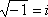“, not “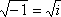“. The i is outside the radical.)

• Simplify sqrt(–25).
• Simplify sqrt(–18).
• Simplify –sqrt(–6).

In your computations, you will deal with i just as you would with x, except for the fact that x2 is just x2, but i2 is –1:

• Simplify 2i + 3i.

2i + 3i = (2 + 3)i = 5i

• Simplify 16i – 5i.

16i – 5i = (16 – 5)i = 11i

• Multiply and simplify (3i)(4i).

(3i)(4i) = (3·4)(i·i) = (12)(i2) = (12)(–1) = –12

• Multiply and simplify (i)(2i)(–3i).

(i)(2i)(–3i) = (2 · –3)(i · i · i) = (–6)(i2 · i)

=(–6)(–1 · i) = (–6)(–i) = 6i

Note this last problem. Within it, you can see that, because i2 = –1. Continuing, we get:

This pattern of powers, signs, 1‘s, and i‘s is a cycle:

In other words, to calculate any high power of i, you can convert it to a lower power by taking the closest multiple of 4 that’s no bigger than the exponent and subtracting this multiple from the exponent. For example, a common trick question on tests is something along the lines of “Simplify i99“, the idea being that you’ll try to multiply i ninety-nine times and you’ll run out of time, and the teachers will get a good giggle at your expense in the faculty lounge. Here’s how the shortcut works:

i99 = i96+3 = i(4×24)+3 = i3 = –i

That is, i99 = i3, because you can just lop off the i96. (Ninety-six is a multiple of four, so i96 is just 1, which you can ignore.) In other words, you can divide the exponent by 4 (using long division), discard the answer, and use only the remainder. This will give you the part of the exponent that you care about. Here are a few more examples:

• Simplify i17.

i17 = i16 + 1 = i4 · 4 + 1 = i1 = i

• Simplify i120.

i120 = i4 · 30 = i4· 30 + 0 = i0 = 1

• Simplify i64,002.

i64,002 = i64,000 + 2 = i4 · 16,000 + 2 = i2 = –1

Now you’ve seen how imaginaries work; it’s time to move on to complex numbers. “Complex” numbers have two parts, a “real” part (being any “real” number that you’re used to dealing with) and an “imaginary” part (being any number with an “i” in it). The “standard” format for complex numbers is “a + bi“; that is, real-part first and i-part last.

Operations on Complex Numbers (page 2 of 3)

Complex numbers are “binomials” of a sort, and are added, subtracted, and multiplied in a similar way. (Division, which is further down the page, is a bit different.) First, though, you’ll probably be asked to demonstrate that you understand the definition of complex numbers.

• Solve 3 – 4i = x + yi

Finding the answer to this involves nothing more than knowing that two complex numbers can be equal only if their real and imaginary parts are equal. In other words, 3 = x and –4 = y.

To simplify complex-valued expressions, you combine “like” terms and apply the various other methods you learned for working with polynomials.

• Simplify (2 + 3i) + (1 – 6i).

(2 + 3i) + (1 – 6i) = (2 + 1) + (3i – 6i) = 3 + (–3i) = 3 – 3i

• Simplify (5 – 2i) – (–4 – i).

(5 – 2i) – (–4 – i)

= (5 – 2i) – 1(–4 – i) = 5 – 2i – 1(–4) – 1(–i)

= 5 – 2i + 4 + i = (5 + 4) + (–2i + i)

= (9) + (–1i) = 9 – i

You may find it helpful to insert the “1” in front of the second set of parentheses (highlighted in red above) so you can better keep track of the “minus” being multiplied through the parentheses.

• Simplify (2 – i)(3 + 4i).

(2 – i)(3 + 4i) = (2)(3) + (2)(4i) + (–i)(3) + (–i)(4i)

= 6 + 8i – 3i – 4i2 = 6 + 5i – 4(–1)

= 6 + 5i + 4 = 10 + 5i

For the last example above, FOILing works for this kind of multiplication, if you learned that method. But whatever method you use, remember that multiplying and adding with complexes works just like multiplying and adding polynomials, except that, while x2 is just x2, i2 is –1. You can use the exact same techniques for simplifying complex-number expressions as you do for polynomial expressions, but you can simplify even further with complexes because i2 reduces to the number –1.

Adding and multiplying complexes isn’t too bad. It’s when you work with fractions (that is, with division) that things turn ugly. Most of the reason for this ugliness is actually arbitrary. Remember back in elementary school, when you first learned fractions? Your teacher would get her panties in a wad if you used “improper” fractions. For instance, you couldn’t say 3/2; you had to convert it to “1 1/2“. But now that you’re in algebra, nobody cares, and you’ve probably noticed that “improper” fractions are often more useful than “mixed” numbers. The issue with complex numbers is that your professor will get his boxers in a bunch if you leave imaginaries in the denominator. So how do you handle this?

• SimplifyThis is pretty “simple”, but they want me to get rid of that i underneath, in the denominator. The 2 in the denominator is fine, but the i has got to go. To do this, I will use the fact that i2 = –1. If I multiply the fraction, top and bottom, by i, then the i underneath will vanish in a puff of negativity:

So the answer isThis was simple enough, but what if they give you something more complicated?

• SimplifyIf I multiply this fraction, top and bottom, by i, I’ll get:

Since I still have an i underneath, this didn’t help much. So how do I handle this simplification? I use something called “conjugates”. The conjugate of a complex number a + bi is the same number, but with the opposite sign in the middle: a – bi. When you multiply conjugates, you are, in effect, multiplying to create something in the pattern of a difference of squares:

Note that the i‘s disappeared, and the final result was a sum of squares. This is what the conjugate is for, and here’s how it is used:

So the answer isIn the last step, note how the fraction was split into two pieces. This is because, technically speaking, a complex number is in two parts, the real part and the i part. They aren’t supposed to “share” the denominator. To be sure your answer is completely correct, split the complex-valued fraction into its two separate terms.

Complex Numbers & The Quadratic Formula (page 3 of 3)

You’ll probably only use complexes in the context of solving quadratics for their zeroes. (There are many other practical uses for complexes, but you’ll have to wait for more interesting classes like “Engineering 201” to get to the “good stuff”.)

Remember that the Quadratic Formula solves “ax2 + bx + c = 0” for the values of x. Also remember that this means that you are trying to find the x-intercepts of the graph. When the Formula gives you a negative inside the square root, you can now simplify that zero by using complex numbers. The answer you come up with is a valid “zero” or “root” or “solution” for “ax2 + bx + c = 0“, because, if you plug it back into the quadratic, you’ll get zero after you simplify. But you cannot graph a complex number on the x,y-plane. So this “solution to the equation” is not an x-intercept. You can make this connection between the Quadratic Formula, complex numbers, and graphing:

 x2 – 2x – 3 x2 – 6x + 9 x2 + 3x + 3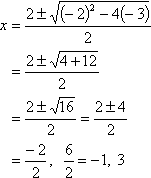a positive number inside the square root zero inside the square root a negative number inside the square root two real solutions one (repeated) real solution two complex solutions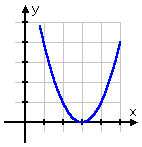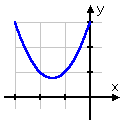two distinct x-intercepts one (repeated) x-intercept no x-intercepts

As an aside, you can graph complexes, but not in the x,y-plane. You need the “complex” plane. For the complex plane, the x-axis is where you plot the real part, and the y-axis is where you graph the imaginary part. For instance, you would plot the complex number 3 – 2i like this:

This leads to an interesting fact: When you learned about regular (“real”) numbers, you also learned about their order (this is what you show on the number line). But x,y-points don’t come in any particular order. You can’t say that one point “comes after” another point in the same way that you can say that one number comes after another number. For instance, you can’t say that (4, 5) “comes after” (4, 3) in the way that you can say that 5 comes after 3. Pretty much all you can do is compare “size”, and, for complex numbers, “size” means “how far from the origin”. To do this, you use the Distance Formula, and compare which complexes are closer to or further from the origin. This “size” concept is called “the modulus”. For instance, looking at our complex number plotted above, its modulus is computed by using the Distance Formula:   Copyright © Elizabeth Stapel 2000-2011 All Rights Reserved

Note that all points at this distance from the origin have the same modulus. All the points on the circle with radius sqrt(13) are viewed as being complex numbers having the same “size” as 3 – 2i.

Every polynomial with complex coefficients can be written as the product of linear factors.